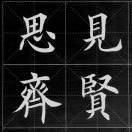V1

2022/03/15阅读：75主题：红绯

# Tikz 作图教程：使用tkz-uclide包作平面图形

Tikz 作图教程：使用tkz-uclide包作平面图形

## 作图步骤

### 1. 定义点

tkz-uclide宏包中定义了一个可以批量定义点的命令——\tkzDefPoints{}，例如\tkzDefPoints{0/0/O,-2.25/-1.5/A,...}, 就可以将 分别储存在字母 O 和 A 中了，以后调用就用 (O),(A)

 \tkzDefPoints{0/0/O, -2.25/-1.5/A, 4/0/B, 0/4/C, 2.2/-1.5/D, 2/3/E, -5/-1.7/F, 3/-1.7/G, 6/1.2/H, -1/1.2/I}

### 2. 连线

tkz-uclide宏包中的连线命令有\tkzDrawSegment []()\tkzDrawSegments[](), 第一个用于作一条线段，第二个用于作折线（多条线段首尾相连）。如果要做一个多边形就用\tkzDrawPolygon[]()来连线。这些命令的小括号中一次放代表点的字母就可以了，字母之间用英文逗号分隔。中括号里放一些属性参数如颜色、虚实线、粗细、箭头等等。

## 文中例子代码

\documentclass[margin=4pt,convert,  convert={    outext=.png,    command=\unexpanded{      pdftocairo -r 600 -png \infile % 将生成的pdf文件转换为png图像    }  },margin=20pt]{standalone}% 支持中文\usepackage{ctex}\usepackage{tkz-euclide}\usepackage{pgfplots}\pgfplotsset{compat=1.17}\begin{document}\begin{tikzpicture}[>=Stealth,scale=2]  \tkzDefPoints{0/0/O, -2.25/-1.5/A, 4/0/B, 0/4/C, 2.2/-1.5/D, 2/3/E, -5/-1.7/F, 3/-1.7/G, 6/1.2/H, -1/1.2/I}  \tkzDrawPolygon[fill=blue!10,draw=blue!15](F,G,H,I)  \draw[->](O)node[below]{$O$}--(A);  \draw[->](O)--(B);  \draw[->](O)--(C)node[left]{$V_2$};  \draw[->,red,thick](O)--node[left]{$\alpha_1$}(D);  \draw[->,red,very thick](O)--node[left]{$\alpha$}(E);  \draw[->,red,thick](D)--node[left]{$\alpha_2$}(E);  \tkzLabelPoint(3,-1){$V_2$} \tkzLabelPoint(-1,-2){$\alpha=\alpha_1+\alpha_2$}; \tkzLabelPoint(-1,-2.4){$V_1\oplus V_2= R^3$}\end{tikzpicture}%\hskip 2cm%\begin{tikzpicture}[>=Stealth,scale=2]\tkzDefPoints{0/0/A, 3.8/0.5/B, 5.6/3.5/C, 2/3/D, 1/6/E, -1/3/F, 2.9/1.75/G, 0.5/3/H, 1/1.5/I, 2.4/3.25/J, 0.5/0.75/K, 1.5/2.25/L, 0/2.25/M, 3.4/2.5/N}\tkzDrawPolygon(A,B,C,D,E,F,A)\tkzDrawSegment[dashed](A,D)\draw[->,red,thick](I)node[right]{$O$}--node[below]{$\alpha_2$}(G);\draw[->,red,thick](I)--node[left]{$\alpha_1$}(H);\draw[->,very thick,red](I)--node[above]{$\alpha$}(J);\draw[red,thick](H)--(J)--(G);\draw[blue,->,thick](I)--node[left]{$\beta$}(K);\draw[blue,->,thick](I)--node[left]{$-\beta$}(L);\draw[orange,->,thick](I)--node[left]{$\alpha_1^\prime$}(M);\draw[orange,->,thick](I)--node[above]{$\alpha_2^\prime$}(N);\draw[orange,thick](M)--(J)--(N);\draw[blue,thick](K)--(M)--(H);\draw[blue,thick](L)--(N)--(G);\tkzLabelPoint(0.8,5.6){$V_1$}\tkzLabelPoint(5,3.3){$V_2$}\tkzLabelPoint(1.2,-0.2){$\alpha=\alpha_1+\alpha_2=\alpha_1^\prime+\alpha_2^\prime$};\tkzLabelPoint(1.2,-0.6){$\alpha_1^\prime=\alpha_1+\beta, \alpha_2^\prime=\alpha_2-\beta$};\tkzLabelPoint(1.2,-1){$V_1+ V_2= R^3$}\end{tikzpicture}\end{document}V1